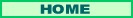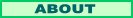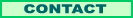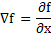## µ (machine understanding) Gradient Covariance

Search

More Tech Stuff:

Indexing Books: Lessons in Language Computations

Client-Side Frame Manipulation Inside the Microsoft Internet Explorer Object Model with Visual Basic .NET

Replacing a PC power supply

Constructing a Mandelbrot Set Based Logo with Visual Basic.NET and Fireworks

Gradients are covariant under a change of coordinate system. That is, there is an equation showing how gradients vary when the scalar values they are calculated from are left in place while a coordinate system change is made. This type of variation is called covariance to distinguish it from contravariance and invariance. Not all mathematical objects fall into one of these three categories. Covariance is an important property with utility in the fields of physics, economics and machine understanding.

In this paper I will work through a relatively simple problem in two dimensions, showing how to derive the gradient from a scalar function, changing the coordinate system, and showing that the transformation of the gradient is covariant. This is not a proof. The specificity of the example may help to understand the general formulas that have long been available in textbooks.  It will also help novices get used to the type of notation used in such problems (for good reason), which differs from notation typically used in high school algebra or introductory calculus courses. The exercise will require ordinary arithmetic, partial derivatives, and matrices.

A gradient is an extension of the concept of slope in introductory algebra and of the derivative in introductory calculus. Instead of thinking of an x and a y axis, with the slope between two points (or at a point) being the change in y divided by the change in x, think of just the x axis. Now to each point of the x axis assign a scalar, which is simply a real value. In mathematics we might use a function to assign these scalar values, as in f(x) = b, a constant along the whole number line, or f(x) = bx, which if the scalars were mapped on a y axis would be a line with a slope of b. In science, in contrast, the scalars might be actual data readings. But here we want the one-dimensional gradient, or change between scalar values.

In one dimension, we could use the full derivative, but since we are headed to multiple dimensions, we might as well get used to the partial derivative. We introduce the partial derivative with respect to x, ∂x, and also the symbol for taking the gradient, ∇. [The symbol is typically pronounced "del," although it is the nabla symbol.] Letting f represent the set of scalar values (the x axis being understood):Except for the one-dimensional case just shown, in which the result is a single-valued scalar, gradients are vectors. In addition, when combined with a transformation law for changes in coordinates, gradients are an example of covariant tensors (which is really what we are working towards). The notation we use is from tensor mathematics, but this case is simple enough it should cause little confusion to a novice. You don’t even need to know what a tensor is to follow this example.

For more than one dimension, we change the notation for the dimensions, or axes, from the familiar x, y, z of high school. We might need a lot of dimensions, so we make them all x’s with subscripts (or even superscripts, but in this paper we’ll stick to subscripts). So our coordinate axes areThis may seem overkill in this example, where I could make do with x and y, but if you are on this road you are heading for higher dimensions, so you might as well learn the lingo.

Given that we have a space of n dimensions, and at every point in the space there is a scalar (a real value), which we will represent by f, the definition of the gradient (at any given point in the space) is: